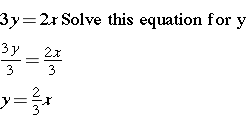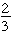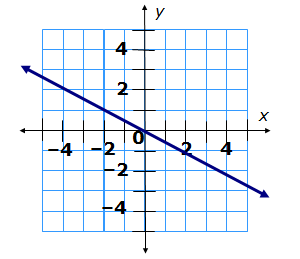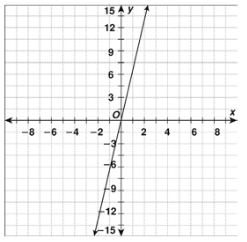# Direct VariationIn this lesson we will learn how to represent proportional relationships using the form y = kx. Basically, this equation represents the relationship between x and y, where one is a constant multiple of the other. In other words, when one variable changes, the other variable changes by a constant factor (k). This constant is known as the constant of proportionality.

There are several ways to represent proportional relationships. They can be represented by a table, a graph, and an equation. You can read direct variation as “y varies directly as x” or “y is directly proportional to x” or “y varies with x.”

It’s simpler when you look at examples.

### Example 1 – Equations

We will begin with direct variation equations. Look at the equations below and determine whether each represents a direct variation. If so, we need to identify the constant of proportionality. (Remember, the direct variation equation is y = kx.)

1.2.This is in the form y = kx and the constant of proportionality is. In other words,is k. This equation is direct variation.

There is another way to quickly see if an equation is direct variation. Plug in 0 for both the x and y values. We are going to try this for the same equations and see what happens. This strategy does not help you find the constant and can only be used for determination.### Example 2 – Function Table

Look at the function tables below. Can you see the pattern? Remember, when you have a table giving you the x and y values, you simply divide y by x to see if you get a constant (K). If you do not get the same value, then the function table does not represent direct variation.

Look at the vertical table below. Divide 8 by 1, 16 by 2, 24 by 3, etc. The answer is all the same. This is an example of direct variation.

y = kx
x y
0 0
1 8
2 16
3 24
4 32
5 40

Tables can be used to write direct variation equations. You divide y by x and get the same value, which will become k. For example, in the direct variation table above, each time you divide y by x, you should get 8. This means that 8 is the constant and that each time x increases by 1, y increases by 8. Once you have this information, you can write an equation. The equation for this table would be: y = 8xx 1 2 3 4 5 6 7 8
y 4 5 6 7 8 9 10 11

This is not an example of direct variation. 4 divided by 1 is not the same as 5 divided by 2.

### Example 3 – Graphs

The graph of a direct variation (proportional relationship) is a straight line that passes through the origin (0,0). On a direct variation graph, the graph will always pass through (1, k), where k is the constant of proportionality. When x is 1, this also represents the unit rate.

Take a look at the graphs below and note differences in the graph that represents direct variation and the graph that does not represent direct variation.Notice that in the first graph the line does not pass through (0, 0). This is not a direct variation.

In the second graph, the graph shows a straight line through (0, 0), so it does represent direct variation (proportional relationship). The Constant of proportionality is, so. Notice that the line goes downward from left to right – which means negative slope. If the constant is positive, the line will slant upward from left to right. An equation can be written from this graph once you find the constant of proportionality. The equation is.

Equations, tables, and graphs may be used to represent proportional relationships or direct variation. You can use any of these representations interchangeably. You can use any of these depictions to solve word problems involving direct variation.

A bicycle travels at a speed of 10 miles per hour. Write a direct variation equation for the distance y the bike travels in hours.   (The constant of proportionality is 10 because each time the bicycle travels 1 hour; it will go another 10 miles.)

y = 10x

A bicycle travels at a speed of 10 miles per hour. Graph the data.

First, make a table. Since our subject is speed, negative numbers will not be used.

y = kx
x hours 1 2 3 4 5 6 7 8
y miles 10 20 30 40 50 60 70 80

Use the ordered pairs to plot the points on a coordinate plane. Connect the points in a straight line. Label each axis.###Videos

1. Here’s a video on the topic of Direct Variation.
2. This video practices graphing.

## Direct Variation Practice

Determine if the table, graph, equation, or word problem represents direct variation (proportional relationship). If yes, identify the constant of proportionality (k).

1. 5y = 60x

4.The line intersects at (0,0), (1,6), (2,12).
5.The link intersects at (0,0), (1, 15), (2, 30).

6.7.

 x 0 1 3 5 y 0 4 12 20

8.

 x 3 9 18 y 1 4 7

9. A pipeline delivers 12,000 gallons of natural gas every other month.

10. An empty swimming pool is being filled at a rate of 5 gallons per minute.

(edited from source)